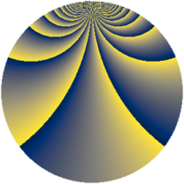# Properties

 Label 1792.2.cbLevel $1792$ Weight $2$ Character orbit 1792.cb Rep. character $\chi_{1792}(37,\cdot)$ Character field $\Q(\zeta_{192})$ Dimension $16256$ Sturm bound $512$

# Learn more

## Defining parameters

 Level: $$N$$ $$=$$ $$1792 = 2^{8} \cdot 7$$ Weight: $$k$$ $$=$$ $$2$$ Character orbit: $$[\chi]$$ $$=$$ 1792.cb (of order $$192$$ and degree $$64$$) Character conductor: $$\operatorname{cond}(\chi)$$ $$=$$ $$1792$$ Character field: $$\Q(\zeta_{192})$$ Sturm bound: $$512$$

## Dimensions

The following table gives the dimensions of various subspaces of $$M_{2}(1792, [\chi])$$.

Total New Old
Modular forms 16512 16512 0
Cusp forms 16256 16256 0
Eisenstein series 256 256 0

## Trace form

 $$16256q - 32q^{2} - 32q^{3} - 32q^{4} - 32q^{5} - 128q^{6} - 64q^{7} - 128q^{8} - 32q^{9} + O(q^{10})$$ $$16256q - 32q^{2} - 32q^{3} - 32q^{4} - 32q^{5} - 128q^{6} - 64q^{7} - 128q^{8} - 32q^{9} - 32q^{10} - 32q^{11} - 32q^{12} - 128q^{13} - 64q^{14} - 128q^{15} - 32q^{16} - 32q^{17} - 32q^{18} - 32q^{19} - 128q^{20} - 64q^{21} - 128q^{22} - 32q^{23} - 32q^{24} - 32q^{25} - 32q^{26} - 128q^{27} - 64q^{28} - 128q^{29} - 32q^{30} - 32q^{31} - 32q^{32} - 32q^{33} - 128q^{34} - 64q^{35} - 128q^{36} - 32q^{37} - 32q^{38} - 32q^{39} - 32q^{40} - 128q^{41} - 64q^{42} - 128q^{43} - 32q^{44} - 32q^{45} - 32q^{46} - 32q^{47} - 128q^{48} - 64q^{49} - 128q^{50} - 32q^{51} - 32q^{52} - 32q^{53} - 32q^{54} - 128q^{55} - 64q^{56} - 128q^{57} - 32q^{58} - 32q^{59} - 32q^{60} - 32q^{61} - 128q^{62} - 64q^{63} - 128q^{64} - 32q^{65} - 32q^{66} - 32q^{67} - 32q^{68} - 128q^{69} - 64q^{70} - 128q^{71} - 32q^{72} - 32q^{73} - 32q^{74} - 32q^{75} - 128q^{76} - 64q^{77} - 128q^{78} - 32q^{79} - 32q^{80} - 32q^{81} - 32q^{82} - 128q^{83} - 64q^{84} - 128q^{85} - 32q^{86} - 32q^{87} - 32q^{88} - 32q^{89} - 128q^{90} - 64q^{91} - 128q^{92} - 32q^{93} - 32q^{94} - 32q^{95} - 32q^{96} - 128q^{97} - 64q^{98} - 128q^{99} + O(q^{100})$$

## Decomposition of $$S_{2}^{\mathrm{new}}(1792, [\chi])$$ into newform subspaces

The newforms in this space have not yet been added to the LMFDB.